Next: Virial Analysis Up: Hydrostatic Balance Previous: Bonnor-Ebert Mass   Contents

## Equilibria of Cylindrical Cloud

In Figure 4.1 (right) we plotted the structure for a polytropic cloud. Inner structure is not dependent of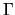, it is clear the slope of the outer envelope is dependent on.

1. In the case of the spherically symmetric, consider a polytrope (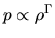) with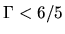(at least the envelope of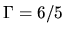cloud extends to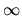.), in which gas extends to. if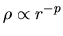, the mass inside of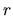is proportional to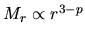. Thus, the gravity per unit volume at a radius,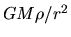, is proportional to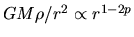. On the other hand the pressure force is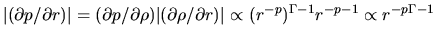. These two powers become the same, only if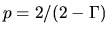.
2. In the case of cylindrical cloud with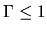, the mass per unit length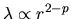. The gravity at,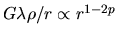. Note that the power is the same as the spherical case. Since the power of the pressure force should be the same as the spherical case, the resultant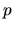should be the same.
The case of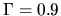, an envelope extending to a large radius indicates the power-law distribution much shallower than that of the isothermal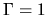one.Next: Virial Analysis Up: Hydrostatic Balance Previous: Bonnor-Ebert Mass   Contents
Kohji Tomisaka 2007-07-08